Question

# 4 PROBABILITY (16) An experiment consists of tossing a fair coin (head and tail T) three...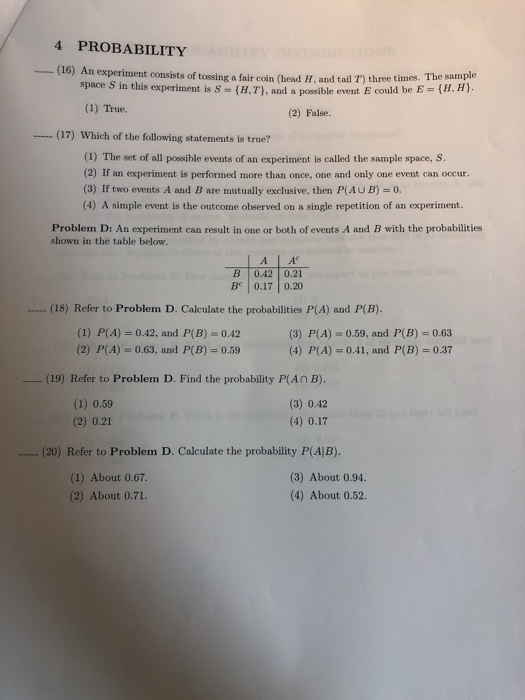4 PROBABILITY (16) An experiment consists of tossing a fair coin (head and tail T) three times. The sample space S in this experiment is S - (HT), and a possible event Ecould be E = {H,H). (1) True. (2) False (17) Which of the following statements is true? (1) The set of all possible events of an experiment is called the sample space, S. (2) If an experiment is performed more than once, one and only one event can occur. (3) If two events A and B are mutually exclusive, then P(AUB) = 0. (4) A simple event is the outcome observed on a single repetition of an experiment. Problem D: An experiment can result in one or both of events A and B with the probabilities shown in the table below. B BC А A 0.42 0.21 0.17 0.20 (18) Refer to Problem D. Calculate the probabilities P(A) and P(B). (1) P(A) = 0.42, and P(B) = 0.42 (3) P(A) - 0.59, and P(B) = 0.63 (2) P(A) = 0.63, and P(B) = 0.59 (4) P(A) = 0.41, and P(B) = 0.37 (19) Refer to Problem D. Find the probability P(ANB). (1) 0.59 (3) 0.42 (2) 0.21 (4) 0.17 (20) Refer to Problem D. Calculate the probability P(AB). (1) About 0.67 (3) About 0.94 (2) About 0.71. (4) About 0.52

(16) false S ={ HHH, HHT , HTH, THH, TTH, HTT, THT ,TTT }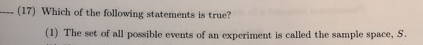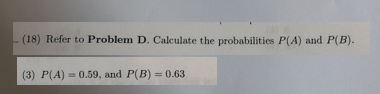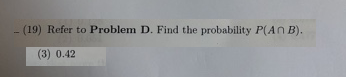(20) P(A/B) = P( A&B) / P(B)

= 0.42/ 0.63

= 2/3 = 0.66667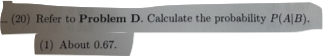--------------------------------------------------------------------------------------

#### Earn Coins

Coins can be redeemed for fabulous gifts.

Similar Homework Help Questions
• ### Problem D: An experiment can result in one or both of events A and B with...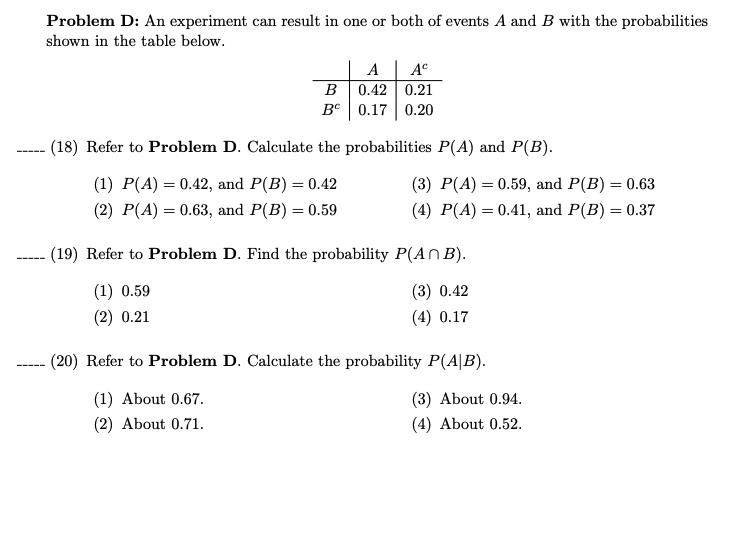Problem D: An experiment can result in one or both of events A and B with the probabilities shown in the table below. A B 0.42 0.21 BC 0.17 0.20 (18) Refer to Problem D. Calculate the probabilities P(A) and P(B). (1) P(A) = 0.42, and P(B) = 0.42 (3) P(A) = 0.59, and P(B) = 0.63 (2) P(A) = 0.63, and P(B) = 0.59 (4) P(A) = 0.41, and P(B) = 0.37 (19) Refer to Problem D. Find the...

• ### An experiment consists of tossing a fair coin (head H, and tail T) three times. The...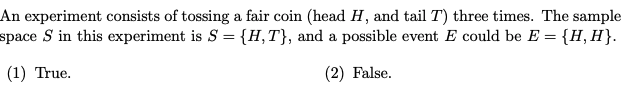An experiment consists of tossing a fair coin (head H, and tail T) three times. The sample space S in this experiment is S = {H, T}, and a possible event E could be E = {H,H}. (1) True. (2) False.

• ### 2. Consider tossing a coin twice. Denote H ="head" and T ="tail" (a) List all outcomes...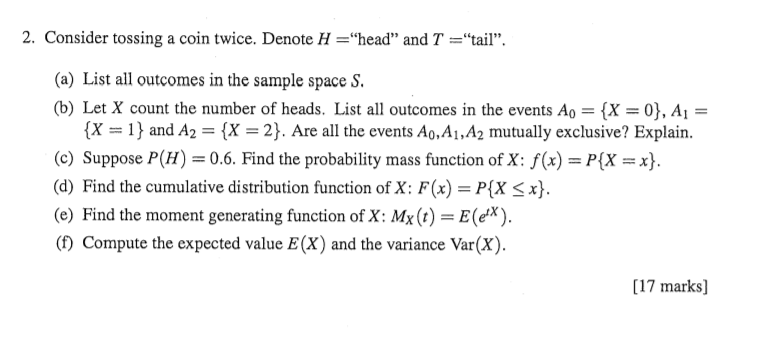2. Consider tossing a coin twice. Denote H ="head" and T ="tail" (a) List all outcomes in the sample space S (b) Let X count the number of heads. List all outcomes in the events Ao = {X = 0}, Ai = {X=1 and A2 {X = 2}. Are all the events Ao,A1,A2 mutually exclusive? Explain. (c) Suppose P(H) = 0.6. Find the probability mass function of X: f(x) = P{X =x} (d) Find the cumulative distribution function of X:...

• ### Example : In tossing a coin once , Find the probability of events ? 1) The...

Example : In tossing a coin once , Find the probability of events ? 1) The event A is the first tossing is heads 2) The event B is the first tossing is tails 3) The event c is the getting is one at least heads 4) The event c is the getting is one at most tails -------------------------------- Example : In tossing a coin once , Find the probability of events ? 1) The event A is the first...

• ### An experiment consists of tossing two dice. a) List all outcomes in the sample space Ω...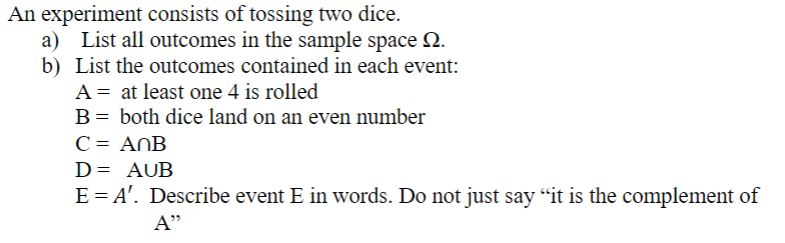An experiment consists of tossing two dice. a) List all outcomes in the sample space Ω b) List the outcomes contained in each event: A- at least one 4 is rolled B-both dice land on an even number D-AUB E A. Describe event E in words. Do not just say "it is the complement of 25

• ### Problem 4, 5 p. ] (in prepation to the binomial model) Consider tossing a coin n...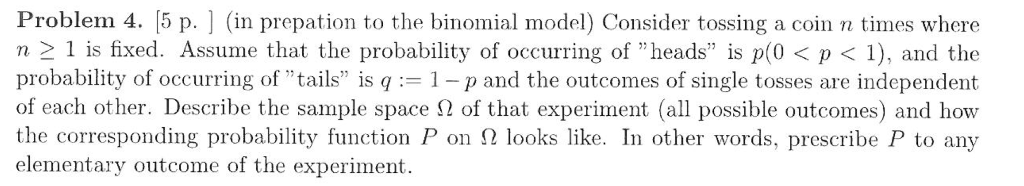Problem 4, 5 p. ] (in prepation to the binomial model) Consider tossing a coin n times where n 1 is fixed. Assume that the probability of occurring of "heads" is p(0< p1), and the probability of occurring of "tails" is q1-p and the outcomes of single tosses are independent of each other. Describe the sample space Ω of that experiment (all possible outcomes) and how the corresponding probability function P on Ω looks like. In other words, prescribe P...

• ### (17) Which of the following statements is true? (1) The set of all possible events of...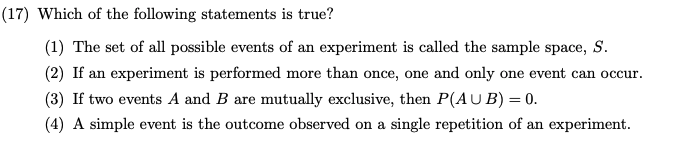(17) Which of the following statements is true? (1) The set of all possible events of an experiment is called the sample space, S. (2) If an experiment is performed more than once, one and only one event can occur. (3) If two events A and B are mutually exclusive, then P(AUB) = 0. (4) A simple event is the outcome observed on a single repetition of an experiment.

• ### PLEASE ANSWER EVERY SINGLE ONE 14. A coin is tossed three times. What is the probability...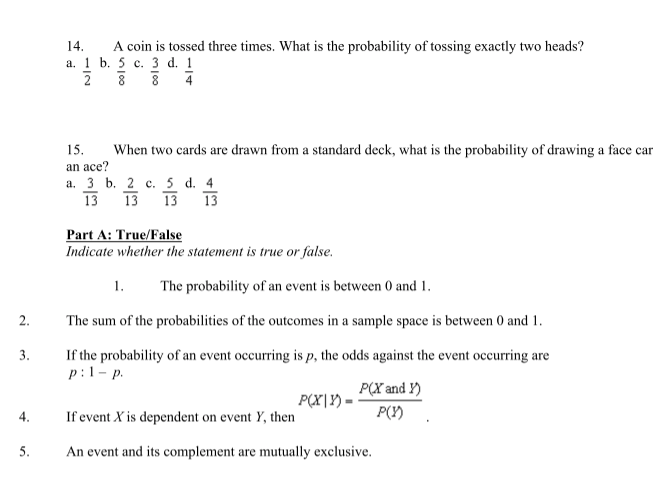PLEASE ANSWER EVERY SINGLE ONE 14. A coin is tossed three times. What is the probability of tossing exactly two heads? a. 1 b. 5 c. 3 d. 1 2 3 3 15. When two cards are drawn from a standard deck, what is the probability of drawing a face car an ace? a. 3 b. 2 c. 5 d. 4 13 13 13 13 Part A: True/False Indicate whether the statement is true or false. 1. The probability of...

• ### 1. Consider a fair four-sided die, with sides 1, 2, 3, and 4, that is rolled...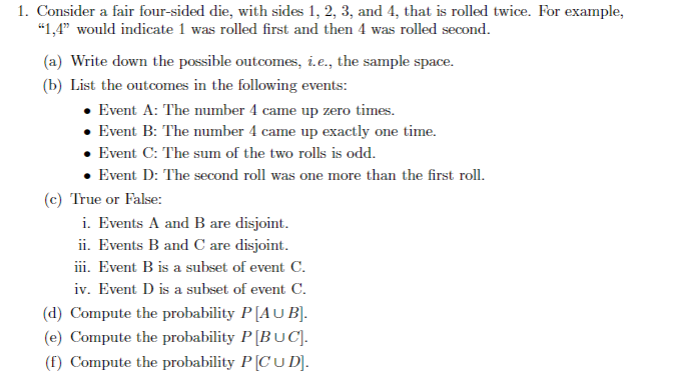1. Consider a fair four-sided die, with sides 1, 2, 3, and 4, that is rolled twice. For example, "1,4" would indicate 1 was rolled first and then 4 was rolled second a) Write down the possible outcomes, i.e., the sample space. (b) List the outcomes in the following events: Event A: The number 4 came up zero times. Event B: The number 4 came up exactly one time. . Event C: The sum of the two rolls is odd...

• ### Show all steps1111111111111111111111111111111111111111111111111111111111111111111111111111111111111111111111111111111111111111111111111111111111111111111111111111 4. Consider the experiment of flipping a coin 5 times and keeping track,...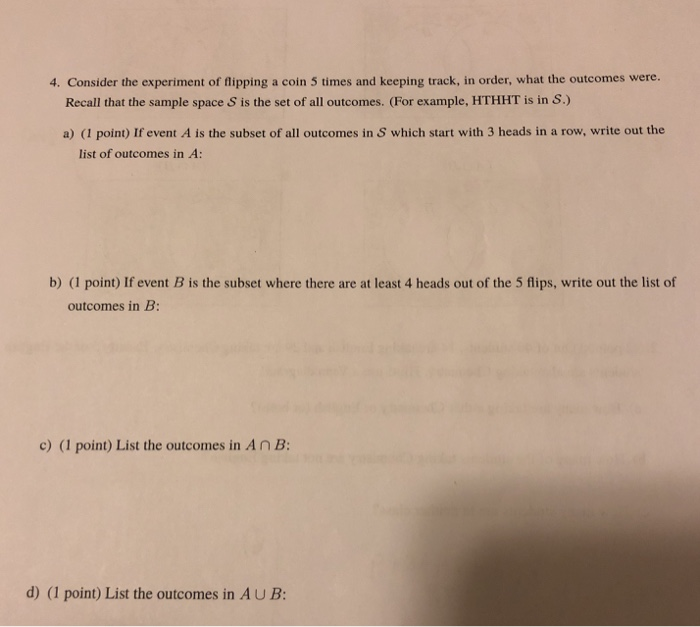Show all steps1111111111111111111111111111111111111111111111111111111111111111111111111111111111111111111111111111111111111111111111111111111111111111111111111111 4. Consider the experiment of flipping a coin 5 times and keeping track, in order, what the outcomes were. Recall that the sample space S is the set of all outcomes. (For example, HTHHT is in S.) a) (I point) If event A is the subset of all outcomes in S which start with 3 heads in a row, write out the list of outcomes in A b) (1 point) If event B is the subset where...